# Subtraction in Columns Practice for Grade 3 Students

Teaching subtraction in columns to third-grade students can be made simple and easy to understand. Here's a step-by-step guide on how to teach column subtraction.

Let's subtract 416 from 559.

## Step 1: set up the problem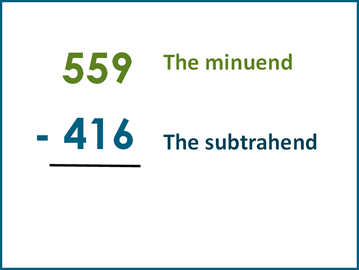## Step 2: start from the right column (ones place)

Subtract the digit in the bottom number (subtrahend) from the digit in the top number (minuend).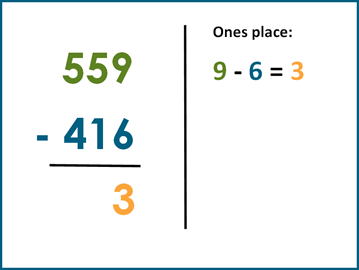## Step 3: move to the tens place

Now, subtract the digit in the tens place of the subtrahend from the digit in the tens place of the minuend.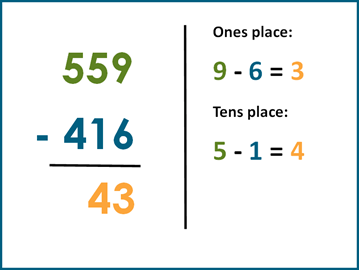## Step 4: finally, move to the hundreds place

Again, subtract the digit in the hundreds place of the subtrahend from the digit in the hundreds place of the minuend.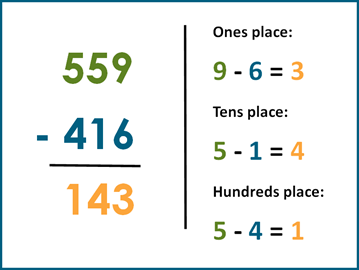Add the difference (143) to the subtrahend (416) to verify that the sum is equal to the minuend (559).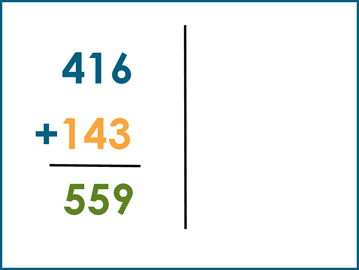## Important points to remember

Borrowing/Regrouping: Sometimes, you'll need to borrow from the next higher place value if the digit on top is smaller than the digit being subtracted from it. Ensure the kids understand this concept.

Place Value: Emphasize the significance of the place value in determining the value to be subtracted.

Practice: Provide plenty of practice problems to reinforce the concept.

Make sure to use various numbers and conduct exercises to solidify their understanding. Visualization aids like base-10 blocks, drawings, or even online interactive tools can be used to make the concept more tangible and engaging for the students.

We have a number of different worksheets for students to practice subtraction in column at the grade 3 level.

## Subtracting 3-digit numbers in columns

In these worksheets students subtract up to 3-digit numbers in columns.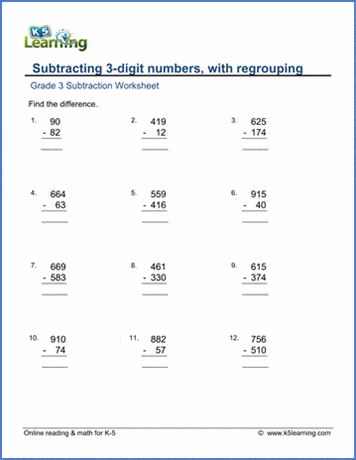## Subtracting 4-digit numbers in columns

In these worksheets students practice subtracting numbers up to 9,999.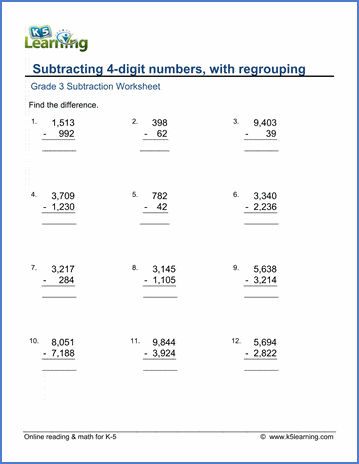## Regrouping across two zeros

These more difficult worksheets involve borrowing across zeros to solve the equations.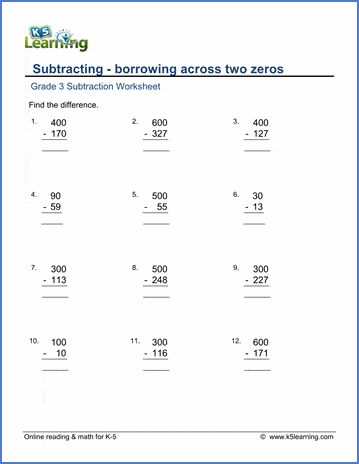## Regrouping across three zeros

These are the most difficult of our subtraction in columns worksheets where students subtract across three zeros.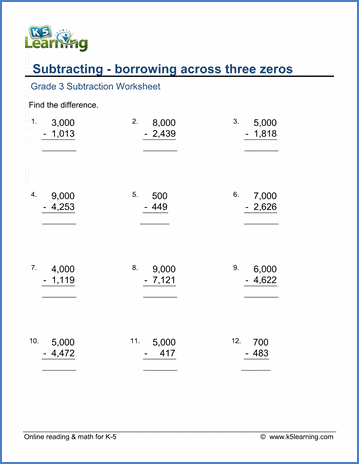Become a Member

This content is available to members only.